Courses

# Computer Science And Information Technology - CS 2013 GATE Paper (Practice Test)

## 65 Questions MCQ Test GATE Past Year Papers for Practice (All Branches) | Computer Science And Information Technology - CS 2013 GATE Paper (Practice Test)

Description
This mock test of Computer Science And Information Technology - CS 2013 GATE Paper (Practice Test) for GATE helps you for every GATE entrance exam. This contains 65 Multiple Choice Questions for GATE Computer Science And Information Technology - CS 2013 GATE Paper (Practice Test) (mcq) to study with solutions a complete question bank. The solved questions answers in this Computer Science And Information Technology - CS 2013 GATE Paper (Practice Test) quiz give you a good mix of easy questions and tough questions. GATE students definitely take this Computer Science And Information Technology - CS 2013 GATE Paper (Practice Test) exercise for a better result in the exam. You can find other Computer Science And Information Technology - CS 2013 GATE Paper (Practice Test) extra questions, long questions & short questions for GATE on EduRev as well by searching above.
QUESTION: 1

### Q. No. 1 – 5 Carry One Mark Each Q. Complete the sentence: Universalism is to particularism as diffuseness is to ________

Solution:

The relation is that of antonyms

QUESTION: 2

Solution:
QUESTION: 3

### Which one of the following options is the closest in meaning to the word given below? Nadir

Solution:

Nadir in the lowest point on a curve

QUESTION: 4

Choose the grammatically INCORRECT sentence:

Solution:
QUESTION: 5

What will be the maximum sum of 44, 42, 40, ... ?

Solution:

The maximum sum is the sum of 44, 42,- - - - -2.
The sum of ‘n’ terms of an AP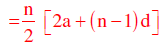In this case, n = 22, a = 2 and d = 2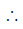Sum =11[4 + 21×2] =11×46 = 506

QUESTION: 6

Q. No. 6 – 10 Carry Two Marks Each

Q.

Out of all the 2-digit integers between 1 and 100, a 2-digit number has to be selected at
random. What is the probability that the selected number is not divisible by 7?

Solution:

The number of 2 digit multiples of 7 = 13Probability of choosing a number
Not divisible by 7 =(90-13)/90 =77/90

QUESTION: 7

A tourist covers half of his journey by train at 60 km/h, half of the remainder by bus at 30
km/h and the rest by cycle at 10 km/h. The average of the tourist in km/h during his entire
journey is

Solution: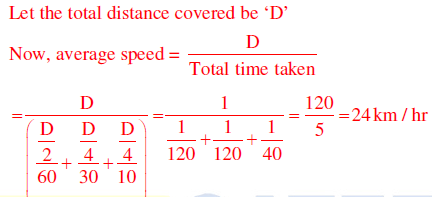QUESTION: 8

Find the sum of the expression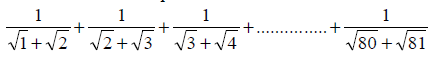Solution: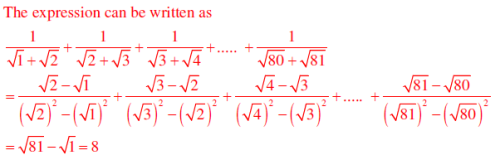QUESTION: 9

The current erection cost of a structure is Rs. 13,200. If the labour wages per day increase by
1/5 of the current wages and the working hours decrease by 1/24 of the current period, then
the new cost of erection in Rs. is

Solution:

Let ‘W’ be the labour wages, and ‘T’ be the working hours.
Now, total cost is a function of W× T
Increase in wages = 20%Revised wages = 1.2 W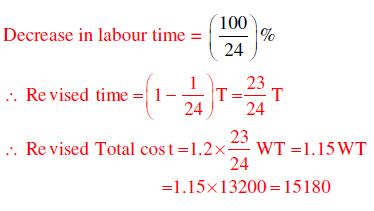QUESTION: 10

After several defeats in wars, Robert Bruce went in exile and wanted to commit suicide. Just
before committing suicide, he came across a spider attempting tirelessly to have its net. Time
and again, the spider failed but that did not deter it to refrain from making attempts. Such
attempts by the spider made Bruce curious. Thus, Bruce started observing the nearimpossible
goal of the spider to have the net. Ultimately, the spider succeeded in having its
net despite several failures. Such act of the spider encouraged Bruce not to commit suicide.
And then, Bruce went back again and won many a battle, and the rest is history.

Which one of the following assertions is best supported by the above information?

Solution:
QUESTION: 11

Q. No. 11 – 35 Carry One Mark Each

Q.

Consider an undirected random graph of eight vertices. The probability that there is an edge
between a pair of vertices is ½. What is the expected number of unordered cycles of length
three?

Solution:

P(edge) =1/2
Number of ways we can choose the vertices out of 8 is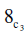(Three edges in each cycle)
Expected number of unordered cycles of length 3 =x (1/2)3 =7

QUESTION: 12

Which of the following statements is/are TRUE for undirected graphs?
P: Number of odd degree vertices is even.
Q: Sum of degrees of all vertices is even.

Solution:

Q: Sum of degrees of all vertices = 2×(number of edges)

QUESTION: 13

Function f is known at the following points: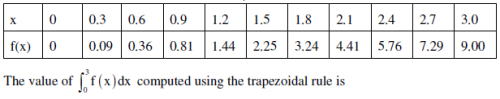Solution: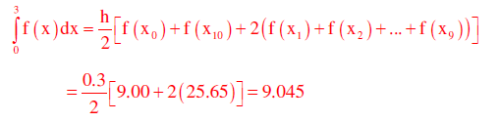QUESTION: 14

Which one of the following functions is continuous at x = 3 ?

Solution: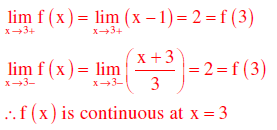QUESTION: 15

Which one of the following expressions does NOT represent exclusive NOR of x and y?

Solution: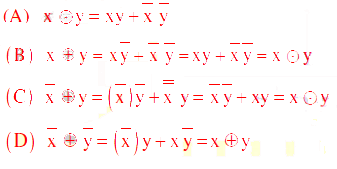QUESTION: 16

In a k-way set associative cache, the cache is divided into v sets, each of which consists of k
lines. The lines of a set are placed in sequence one after another. The lines in set s are
sequenced before the lines in set (s+1). The main memory blocks are numbered 0 onwards.
The main memory block numbered j must be mapped to any one of the cache lines from

Solution:

Position of main memory block in the cache (set) = (main memory block number) MOD
(number of sets in the cache).
As the lines in the set are placed in sequence, we can have the lines from 0 to (K – 1) in each
set.
Number of sets = v, main memory block number = j
First line of cache = (j mod v)*k; last line of cache = (j mod v)*k + (k – 1)

QUESTION: 17

What is the time complexity of Bellman-Ford single-source shortest path algorithm on a
complete graph of n vertices?

Solution: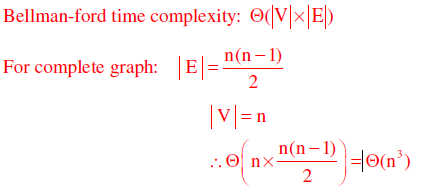QUESTION: 18

Which of the following statements are TRUE?
(1) The problem of determining whether there exists a cycle in an undirected graph is in P.
(2) The problem of determining whether there exists a cycle in an undirected graph is in NP.
(3) If a problem A is NP-Complete, there exists a non-deterministic polynomial time
algorithm to solve A.

Solution:

1. Cycle detection using DFS:  O(V+ E) = O(V2) and it is polynomial problem
2. Every P-problem is NP(since P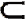NP)
3. NP − complete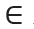NP
Hence, NP-complete can be solved in non-deterministic polynomial time

QUESTION: 19

Which of the following statements is/are FALSE?
(1) For every non-deterministic Turing machine, there exists an equivalent deterministic
Turing machine.
(2) Turing recognizable languages are closed under union and complementation.
(3) Turing decidable languages are closed under intersection and complementation
(4) Turing recognizable languages are closed under union and intersection.

Solution:

(1) NTM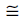DTM
(2) RELs are closed under union & but not complementation
(3) Turing decidable languages are recursive and recursive languages are closed under
intersection and complementation
(4) RELs are closed under union & intersection but not under complementation

QUESTION: 20

Three concurrent processes X, Y, and Z execute three different code segments that access and
update certain shared variables. Process X executes the P operation (i.e., wait) on semaphores
a, b and c; process Y executes the P operation on semaphores b, c and d; process Z executes
the P operation on semaphores c, d, and a before entering the respective code segments. After
completing the execution of its code segment, each process invokes the V operation (i.e.,
signal) on its three semaphores. All semaphores are binary semaphores initialized to one.
Which one of the following represents a deadlock-free order of invoking the P operations by
the processes?

Solution:

Suppose X performs P(b) and preempts, Y gets chance, but cannot do its first wait i.e., P(b),
so waits for X, now Z gets the chance and performs P(a) and preempts, next X gets chance.
X cannot continue as wait on ‘a’ is done by Z already, so X waits for Z. At this time Z can
continue its operations as down on c and d. Once Z finishes, X can do its operations and so Y.
In any of execution order of X, Y, Z one process can continue and finish, such that waiting is
not circular. In options (A),(C) and (D) we can easily find circular wait, thus deadlock

QUESTION: 21

An index is clustered, if

Solution:

Clustered index is built on ordering non key field and hence if the index is clustered then the
data records of the file are organized in the same order as the data entries of the index.

QUESTION: 22

Assume that source S and destination D are connected through two intermediate routers
labeled R. Determine how many times each packet has to visit the network layer and the data
link layer during a transmission from S to D.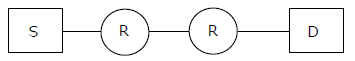Solution: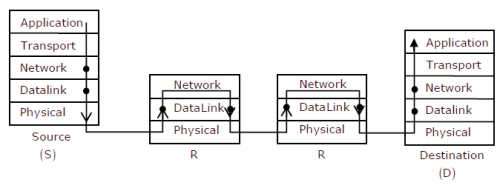From above given diagram, its early visible that packet will visit network layer 4 times, once
at each node [S, R, R, D] and packet will visit Data Link layer 6 times. One time at S and one
time at D, then two times for each intermediate router R as data link layer is used for link to
link communication.
Once at packet reaches R and goes up from physical –DL-Network and second time when
packet coming out of router in order Network – DL- Physical

QUESTION: 23

The transport layer protocols used for real time multimedia, file transfer, DNS and
email, respectively are

Solution:

Real time multimedia needs connectionless service, so under lying transport layer protocol
used is UDP
File transfer rums over TCP protocol with port no-21
DNS runs over UDP protocol within port no-53
Email needs SMTP protocol which runs over TCP protocol within port no – 25

QUESTION: 24

Using public key cryptography, X adds a digital signature σ to message M, encrypts <M,
σ >, and sends it to Y, where it is decrypted. Which one of the following sequences of keys is
used for the operations?

Solution: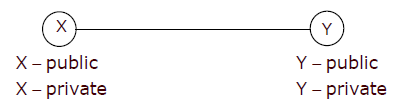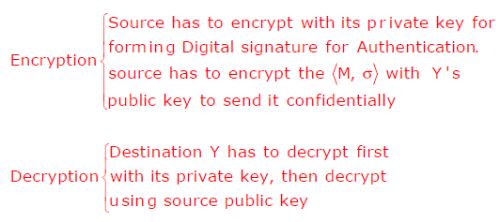QUESTION: 25

Match the problem domains in Group I with the solution technologies in Group II.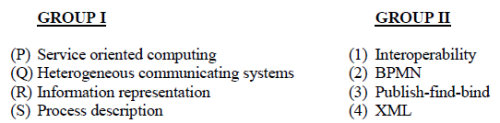Solution:
QUESTION: 26

A scheduling algorithm assigns priority proportional to the waiting time of a process. Every
process starts with priority zero(the lowest priority). The scheduler re-evaluates the process
priorities every T time units and decides the next process to schedule. Which one of the
following is TRUE if the processes have no I/O operations and all arrive at time zero?

Solution:

The given scheduling definition takes two parameters, one is dynamically assigned process
priority and the other is ‘T’ time unit to re-evaluate the process priorities.
This dynamically assigned priority will be deciding processes order in ready queue of round
robin algorithm whose time quantum is same as ‘T’ time units. As all the processes are
arriving at the same time, they will be given same priority but soon after first ‘T’ time burst
remaining processes will get higher priorities

QUESTION: 27

What is the maximum number of reduce moves that can be taken by a bottom-up parser for a
grammar with no epsilon- and unit-production (i.e., of type A →and A→a) to parse a
string with n tokens?

Solution:

To have maximum number of reduce moves, all the productions will be of the typeA→αb
(where  and  could be terminals or non-terminals). Consider the following illustration
then: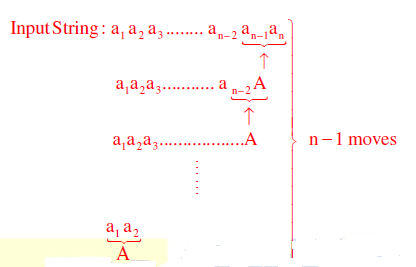QUESTION: 28

Consider the languages and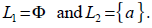. Which one of the following represents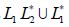?

Solution: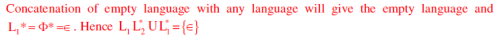QUESTION: 29

Which one of the following is the tightest upper bound that represents the time complexity of
inserting an object into a binary search tree of n nodes?

Solution:

For skewed binary search tree on n nodes, the tightest upper bound to insert a node is O(n)

QUESTION: 30

Which one of the following is the tightest upper bound that represents the number of swaps
required to sort n numbers using selection sort?

Solution:

The maximum number of swaps that takes place in selection sort on n numbers is n

QUESTION: 31

In the following truth table, V = 1 if and only if the input is valid.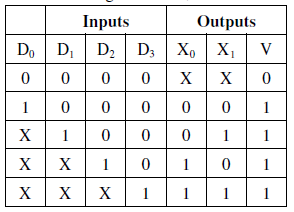What function does the truth table represent?

Solution:

4 to 2 priority encoder.

QUESTION: 32

The smallest integer than can be represented by an 8-bit number in 2’s complement form is

Solution:

−28−1 = −128 . Range is -2(n-1) to +2(n-1)-1

QUESTION: 33

Which one of the following does NOT equal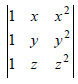Solution:

If matrix B is obtained from matrix A by replacing the lth row by itself plus k times the mth row, for l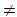m then det(B)=det(A). With this property given matrix is equal to the matrices
given in options (B),(C) and (D).

QUESTION: 34

Suppose p is number of cars per minute passing through a certain road junction between 5
PM and 6PM, and p has a Poisson distribution with mean 3. What is the probability of
observing fewer than 3 cars during any given minute in this interval?

Solution: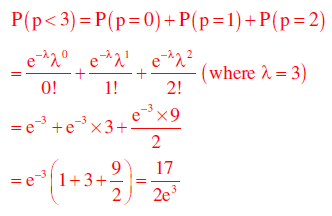QUESTION: 35

A binary operation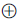on a set of integers is defined as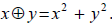. Which one of the
following statements is TRUE about?

Solution:QUESTION: 36

Q. No. 36 – 65 Carry Two Marks Each

Q.

Which one of the following is NOT logically equivalent to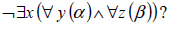Solution: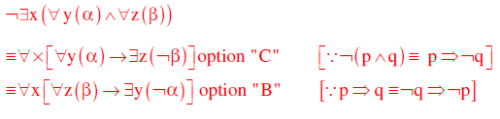QUESTION: 37

A RAM chip has a capacity of 1024 words of 8 bits each (1K x 8) . The number of 2 x 4
decoders with enable line needed to construct a 16K x 16 RAM from1K x 8 RAM is

Solution:

RAM chip size = 1k ×8[1024 words of 8 bits each]
RAM to construct =16k ×16
Number of chips required = (16K x 16)/16 x 2 [16 chips vertically with each having 2 chips horizontally]
So to select one chip out of 16 vertical chips, we need 4 x 16 decoder.
Available decoder is – 2 x 4 decoder
To be constructed is 4 x 16 decoder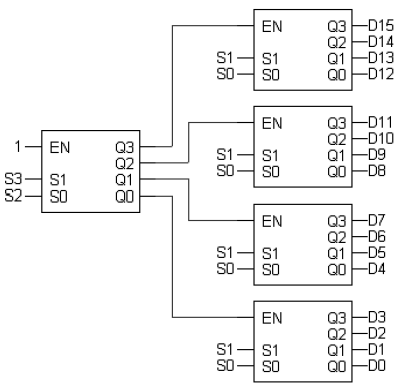So we need 5, 2 x 4 decoder in total to construct 4 x 16 decoder.

QUESTION: 38

Consider an instruction pipeline with five stages without any branch prediction: Fetch
Instruction (FI), Decode Instruction (DI), Fetch Operand (FO), Execute Instruction (EI) and
Write Operand (WO). The stage delays for FI, DI, FO, EI and WO are 5 ns, 7 ns, 10 ns, 8 ns
and 6 ns, respectively. There are intermediate storage buffers after each stage and the delay of
each buffer is 1 ns. A program consisting of I2 instructions  I1 , I2 , I3 ,......I is executed in this
pipelined processor. Instruction  I4 is the only branch instruction and its branch target is 9 I . If
the branch is taken during the execution of this program, the time (in ns) needed to complete
the program is

Solution:

Clock period=Maximum stage delay+ overhead (Buffer) =10+1=11 ns
Assume FI-1, DI-2, FO-3, EI-4, WO-5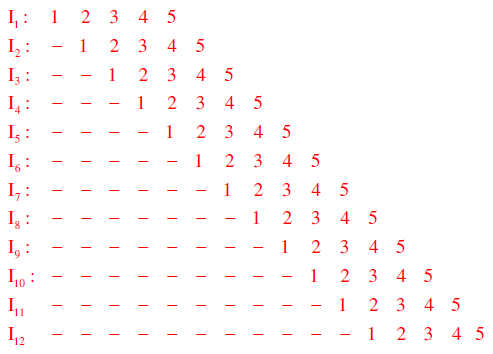So number of clocks required to complete the program is = 15 clocks and time taken is = 15 ×11 ns=165 ns.

QUESTION: 39

Consider the following operation along with Enqueue and Dequeue operations on queues,
where k is a global parameter

MultiDequeue Q {
m k
while Q is not empty and m 0 {
Dequeue Q
m m 1
}
}

What is the worst case time complexity of a sequence of n queue operations on an initially
empty queue?

Solution:

Initially the queue is empty and we have to perform n operations.
i) One option is to perform all Enqueue operations i.e. n Enqueue operations. Complexity
will be (n)
or
ii) We can perform a mix of Enqueue and Dequeue operations. It can be Enqueue for first
n/2 times and then Dequeue for next n/2, or Enqueue and Dequeue alternately, or any
permutation of Enqueues and Dequeues totaling ‘n’ times. Complexity will be (n)
or
iii) We can perform Enqueues and MultiDequeues. A general pattern could be as follows:

Enqueue Enqueue … (ktimes) MultiDequeue Enqueue Enqueue … (ktimes) MultiDequeue
… Up to total n
---- k items enqueued -----k items deleted----k items enqueued----k items deleted -- and
so on.
The number of times this k-Enqueues, MutiDequeue cycle is performed = n/k +1
So, Complexity will be k times Enqueue + 1 MultiDequeue) x n/k+1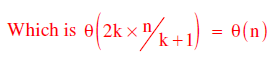or
iv) We can just perform n MultiDequeues (or n Dequeues for that matter):
Each time the while condition is false (empty queue), condition is checked just once for
each of the ‘n’ operations. So θ(n).

QUESTION: 40

The preorder traversal sequence of a binary search tree is 30, 20, 10, 15, 25, 23, 39, 35, 42.
Which one of the following is the postorder traversal sequence of the same tree?

Solution:

Pr eorder :30,20,10,15,25,23,39,35,42
Inorder :10,15,20,23,25,30,35,39,42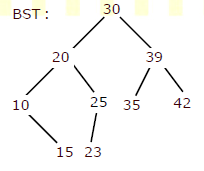QUESTION: 41

What is the return value of  f(p,p) if the value of p is initialized to 5 before the call? Note
that the first parameter is passed by reference, whereas the second parameter is passed by
value.
int f (int &x, int c) {
c= c- 1;
if (c= 0) return 1;
x = x+ 1;
return f (x,c )* x;
}

Solution: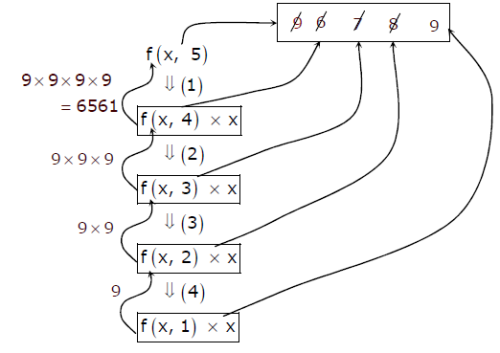QUESTION: 42

Which of the following is/are undecidable?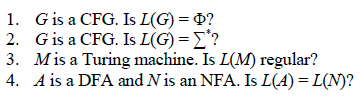Solution:

There is an algorithm to check whether the given CFG is empty, finite or infinite and also to convert NFA to DFA hence 1 and 4 are decidable

QUESTION: 43

Consider the following two sets of LR(1) items of an LR(1) grammar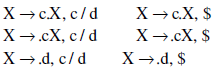Which of the following statements related to merging of the two sets in the corresponding
LALR parser is/are FALSE?

1.  Cannot be merged since look aheads are different
2. Can be merged but will result in S–R conflict
3. Can be merged but will result in R–R conflict
4. Cannot be merged since goto on c will lead to two different sets

Solution: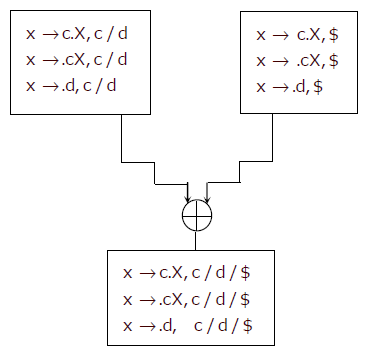1. Merging of two states depends on core part (production rule with dot operator), not on look aheads.
2. The two states are not containing Reduce item ,So after merging, the merged state can not contain any S-R conflict
3. As there is no Reduce item in any of the state, so can’t have R-R conflict.
4. Merging of stats does not depend on further goto on any terminal.
So all statements are false.

QUESTION: 44

A certain computation generates two arrays a and b such that a[i] = f (i) for 0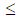i < n and
b[i] = g (a [i]) for 0i < n . Suppose this computation is decomposed into two concurrent
processes X and Y such that X computes the array a and Y computes the array b. The
processes employ two binary semaphores R and S, both initialized to zero. The array a is
shared by the two processes. The structures of the processes are shown below.

Process X:
private i;
for (i=0; i<n; i++) {
a[i] = f(i);
ExitX(R, S);
}

Process Y:
private i;
for (i=0; i<n; i++) {
EntryY(R, S);
b[i] = g(a[i]);
}

Which one of the following represents the CORRECT implementations of ExitX and EntryY?

Solution:

For computing both the array a[] and b[], first element a[i] should be computed using which b[i] can be computed. So process X and Y should run in strict alteration manner, starting with X. This requirement meets with implementation of ExitX and EntryY given in option C.

QUESTION: 45

The following figure represents access graphs of two modules M1 and M2. The filled circles
represent methods and the unfilled circles represent attributes. IF method m is moved to
module M2 keeping the attributes where they are, what can we say about the average
cohesion and coupling between modules in the system of two modules?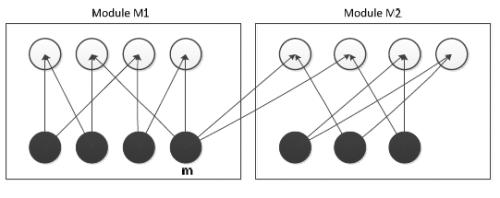Solution: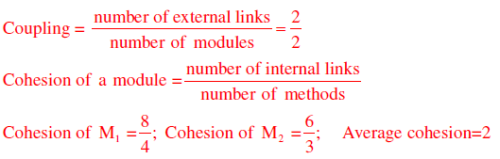After moving method m to M2, graph will become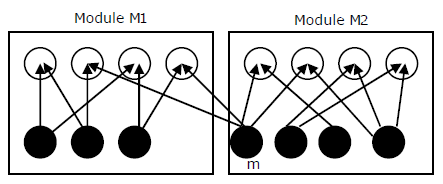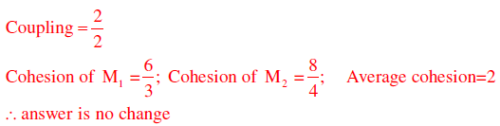QUESTION: 46

In an IPv4 datagram, the M bit is 0, the value of HLEN is 10, the value of total length is 400
and the fragment offset value is 300. The position of the datagram, the sequence numbers of
the first and the last bytes of the payload, respectively are

Solution:

M= 0 – Means there is no fragment after this, i.e. Last fragment
HLEN=10 - So header length is 4×10=40, as 4 is constant scale factor
Total Length = 400(40 Byte Header + 360 Byte Payload)
Fragment Offset = 300, that means 300×8 Byte = 2400 bytes are before this last fragment
So the position of datagram is last fragment
Sequence number of First Byte of Payload = 2400 (as 0 to 2399 Sequence no are used)
Sequence number of Last Byte of Payload = 2400+360-1=2759

QUESTION: 47

Determine the maximum length of cable (in km) for transmitting data at a rate of 500 Mbps in
an Ethernet LAN with frames of size 10,000 bits. Assume the signal speed in the cable to be
2,00,000 km/s

Solution: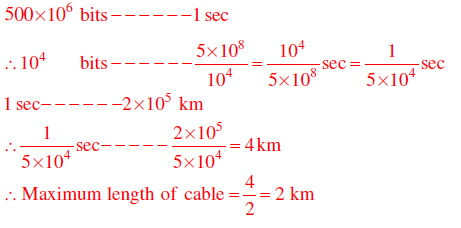QUESTION: 48

Consider the following relational schema.
Students(rollno: integer, sname: string)
Courses(courseno: integer, cname: string)
Registration(rollno: integer, courseno; integer, percent: real)
Which of the following queries are equivalent to this query in English?
“Find the distinct names of all students who score more than 90% in the course numbered
107”
(I) SELECT DISTINCT S.sname
FROM Students as S, Registration as R
WHERE R.rollno=S.rollno AND R.Courseno=107 AND R.percent>90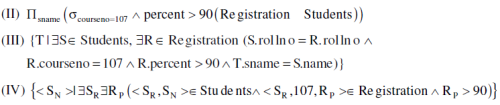Solution:

Four queries given in SQL, RA, TRC and DRC in four statements respectively retrieve the required information.

QUESTION: 49

A shared variable x, initialized to zero, is operated on by four concurrent processes W, X, Y, Z as
follows. Each of the processes W and X reads x from memory, increments by one, stores it to
memory, and then terminates. Each of the processes Y and Z reads x from memory, decrements by
two, stores it to memory, and then terminates. Each process before reading x invokes the P
operation (i.e., wait) on a counting semaphore S and invokes the V operation (i.e., signal) on the
semaphore S after storing x to memory. Semaphore S is initialized to two. What is the maximum
possible value of x after all processes complete execution?

Solution: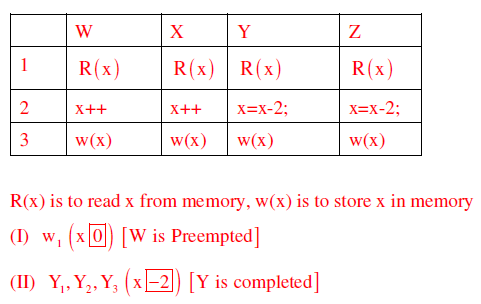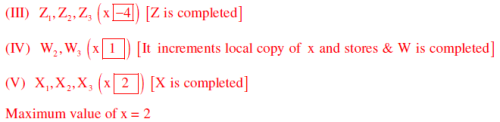QUESTION: 50

Consider the DFA given below.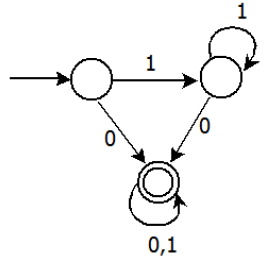Which of the following are FALSE?

1. Complement of L(A) is context–free

2.  L(A) = L((11* 0 + 0)(0 +1)* 0 *1*)
3. For the language accepted by A, A is the minimal DFA
4. A accepts all strings over {0, 1} of length at least 2

Solution:

(1) L(A) is regular, its complement is also regular and if it is regular it is also context free.
(2) L(A) =(11*0 + 0)(0 +1)* 0*1* = 1*0 (0 +1)*
Language has all strings where each string contains ‘0’.
(3) A is not minimal, it can be constructed with 2 states
(4) Language has all strings, where each string contains ‘0’. (atleast length one)

QUESTION: 51

Consider the following languages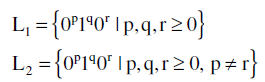Which one of the following statements is FALSE?

Solution: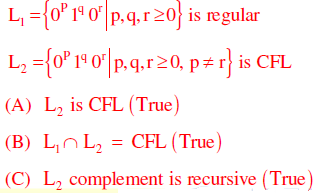(D) L1 complement is CFL but not regular False as L1 is regular L1 is regular

QUESTION: 52

Consider the following function

int unknown (int n) {
int i, j, k 0;
for (i =n/2; i<= n; i++)
for (j= 2; j<= n; j= j* 2)
k= k+ n / 2;
return k ;
}
The return value of the function is

Solution: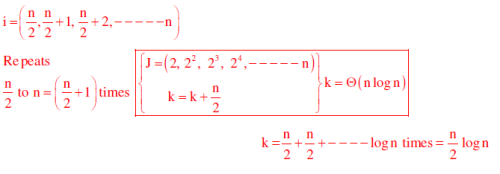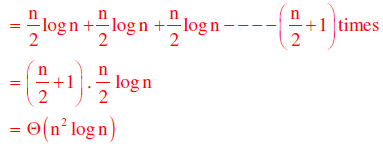QUESTION: 53

The number of elements that can be sorted in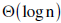time using heap sort is

Solution:

After constructing a max-heap in the heap sort , the time to extract maximum element and
then heapifying the heap takes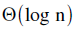time by which we could say thattime is
required to correctly place an element in sorted array. Iftime is taken to sort using
heap sort, then number of elements that can be sorted is constant which is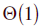QUESTION: 54

Consider a hard disk with 16 recording surfaces (0 −15) having 16384 cylinders (0 −16383)
and each cylinder contains 64 sectors (0 − 63) . Data storage capacity in each sector is 512
bytes. Data are organized cylinder–wise and the addressing format is <cylinder no., sector
no.>. A file of size 42797 KB is stored in the disk and the starting disk location of the file is
<1200, 9, 40>. What is the cylinder number of the last sector of the file, if it is stored in a
contiguous manner?

Solution:

42797 KB=(42797x 1024 )/512= 85594 sectors

Starting is 1200,9,40 contains total 24 + (6×64) = 408 sectors
Next, 1201, --------, 1283 cylinders contains total 1024×83 = 84992 sec tors
(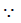each cylinder contains 16 64 1024 sec tors)Total 408 + 84992= 85400 sec torsThe required cylinder number is 1284 which will contain the last sector of the file

QUESTION: 55

Consider the following sequence of micro–operations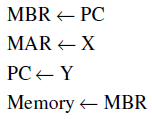Which one of the following is a possible operation performed by this sequence?

Solution:

PC content is stored in memory via MBR and PC gets new address from Y. It represents a
function call (routine), which is matching with interrupt service initiation

QUESTION: 56

The line graph L(G) of a simple graph G is defined as follows:
• There is exactly one vertex v(e) in L(G) for each edge e in G.
• For any two edges e and e’ in G, L(G) has an edge between v(e) and v(e’), if and only if e
and e’ are incident with the same vertex in G.
Which of the following statements is/are TRUE?

(P) The line graph of a cycle is a cycle.
(Q) The line graph of a clique is a clique.
(R) The line graph of a planar graph is planar.
(S) The line graph of a tree is a tree.

Solution:

P) The line graph of a cycle is a cycle

R) Line graph of planar graph need not be planar always. Consider the following example. Consider the following planar graph (star graph)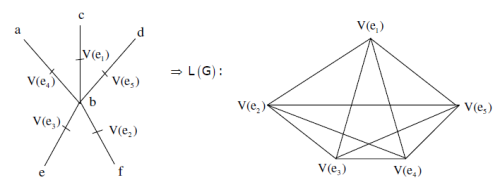S) Hence line graph of planar graph need not be planar(Here we got K5 which is not planar).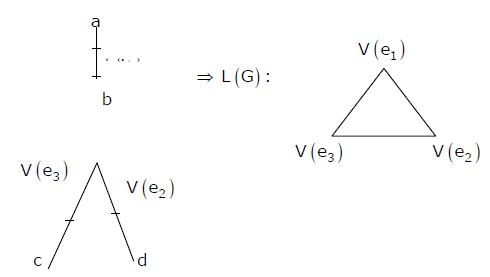The line graph of a tree need not be tree.

QUESTION: 57

What is the logical translation of the following statement?
“None of my friends are perfect.”

Solution:
QUESTION: 58

The procedure given below is required to find and replace certain characters inside an input
character string supplied in array A. The characters to be replaced are supplied in array oldc,
while their respective replacement characters are supplied in array newc. Array A has a fixed
length of five characters, while arrays oldc and newc contain three characters each. However, the
procedure is flawed

void find _ and _ replace (char *A, char *oldc, char * newc ) {
for (int i= 0; i <5; i++)
for (int j =0; j <3; j++)
if (A [i] ==oldc[ j])  A [i]= newc [j] ;
}
The procedure is tested with the following four test cases
(1) oldc = "abc", newc = "dab"            (2) oldc = "cde", newc = "bcd"
(3) oldc = "bca", newc = "cda"            (4) oldc = "abc", newc = "bac"

Q.

The tester now tests the program on all input strings of length five consisting of characters
‘a’, ‘b’, ‘c’, ‘d’ and ‘e’ with duplicates allowed. If the tester carries out this testing with the
four test cases given above, how many test cases will be able to capture the flaw?

Solution:

Flaw in this given procedure is that one character of Array ‘A’ can be replaced by more than one character of newc array, which should not be so.Test case (3) and (4) identifies this flaw as they are containing ‘oldc’ and ‘newc’ array characters arranged in specific manner.
Following string can reflect flaw, if tested by test case (3). initially i = j = 0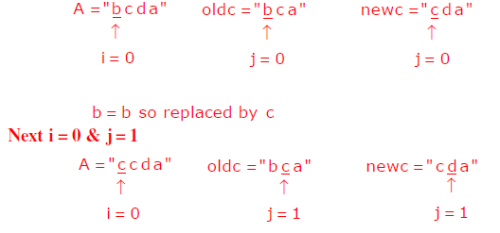c = c so replaced by d

Likewise single character ‘b’ in A is replaced by ‘c’ and then by ‘d’.
Same way test case (4) can also catch the flaw

QUESTION: 59

The procedure given below is required to find and replace certain characters inside an input
character string supplied in array A. The characters to be replaced are supplied in array oldc,
while their respective replacement characters are supplied in array newc. Array A has a fixed
length of five characters, while arrays oldc and newc contain three characters each. However, the
procedure is flawed

void find _ and _ replace (char *A, char *oldc, char * newc ) {
for (int i= 0; i <5; i++)
for (int j =0; j <3; j++)
if (A [i] ==oldc[ j])  A [i]= newc [j] ;
}
The procedure is tested with the following four test cases
(1) oldc = "abc", newc = "dab"            (2) oldc = "cde", newc = "bcd"
(3) oldc = "bca", newc = "cda"            (4) oldc = "abc", newc = "bac"

Q.

If array A is made to hold the string “abcde”, which of the above four test cases will be
successful in exposing the flaw in this procedure?

Solution:

Now for string “abcde” in array A, both test case (3) and (4) will be successful in finding the
flaw, as explained in above question.

QUESTION: 60

The following code segment is executed on a processor which allows only register operands in
its instructions. Each instruction can have almost two source operands and one destination operand.
Assume that all variables are dead after this code segment

c= a+ b;
d = c * a;
e= c+ a;
x =c *c;
if ( x >a) {
y= a * a;
}
else {
d =d * d;
e =e * e;
}

Q.

Suppose the instruction set architecture of the processor has only two registers. The only allowed
compiler optimization is code motion, which moves statements from one place to another while
preserving correctness. What is the minimum number of spills to memory in the compiled code?

Solution:
QUESTION: 61

The following code segment is executed on a processor which allows only register operands in
its instructions. Each instruction can have almost two source operands and one destination operand.
Assume that all variables are dead after this code segment

c= a+ b;
d = c * a;
e= c+ a;
x =c *c;
if ( x >a) {
y= a * a;
}
else {
d =d * d;
e =e * e;
}

Q.

What is the minimum number of registers needed in the instruction set architecture of the
processor to compile this code segment without any spill to memory? Do not apply any
optimization other than optimizing register allocation

Solution: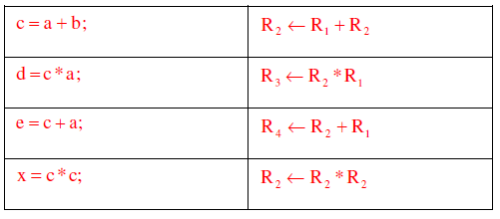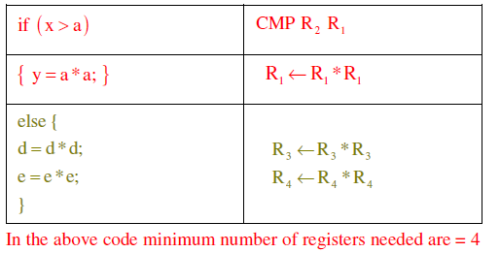QUESTION: 62

Relation R has eight attributes ABCDEFGH. Fields of R contain only atomic values.
F ={CH→G, A→BC, B→CFH, E→A, F→EG} is a set of functional dependencies (FDs) so that F+ is exactly the set of FDs that hold for R

Q.

How many candidate keys does the relation R have?

Solution:

Candidate keys are AD, BD, ED and FD

QUESTION: 63

Relation R has eight attributes ABCDEFGH. Fields of R contain only atomic values.
F ={CH→G, A→BC, B→CFH, E→A, F→EG} is a set of functional dependencies (FDs) so that F+ is exactly the set of FDs that hold for R

Q.

The relation R is

Solution:

A→BC,B→CFH and F→EG are partial dependencies. Hence it is in 1NF but not in 2NF

QUESTION: 64

A computer uses 46–bit virtual address, 32–bit physical address, and a three–level paged page
table organization. The page table base register stores the base address of the first–level table
( T1) ,which occupies exactly one page. Each entry of T1 stores the base address of a page of the
second–level table ( T2) . Each entry of T2 stores the base address of a page of the third–level table
( T3)  Each entry of T3 stores a page table entry (PTE). The PTE is 32 bits in size. The processor
used in the computer has a 1 MB 16 way set associative virtually indexed physically tagged cache.
The cache block size is 64 bytes.

Q.

What is the size of a page in KB in this computer?

Solution: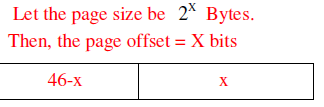Now, we are using 3-level paging. First level page table is contained in one page. Each page table entry is 32-bit.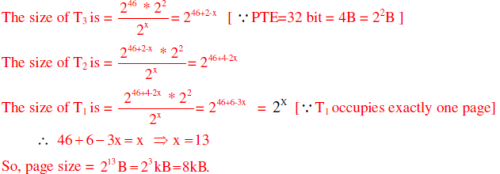QUESTION: 65

A computer uses 46–bit virtual address, 32–bit physical address, and a three–level paged page
table organization. The page table base register stores the base address of the first–level table
( T1) ,which occupies exactly one page. Each entry of T1 stores the base address of a page of the
second–level table ( T2) . Each entry of T2 stores the base address of a page of the third–level table
( T3)  Each entry of T3 stores a page table entry (PTE). The PTE is 32 bits in size. The processor
used in the computer has a 1 MB 16 way set associative virtually indexed physically tagged cache.
The cache block size is 64 bytes.

Q.

What is the minimum number of page colours needed to guarantee that no two synonyms map to
different sets in the processor cache of this computer?

Solution:

As the page size is  213 Bytes and page coloring is asked so we divide cache size by page size and group 16 pages in one set.
Number of pages in cache=1MB/8KB=128 pages

Number of set in cache=128/16=8 sets

Take any page of LAS, it will be mapped with cache on any one of these 8 sets (set
association mapping).For any two synonym to map with same set they should be colored with same color of that respective set. So minimum we need 8 colors for this mapping.